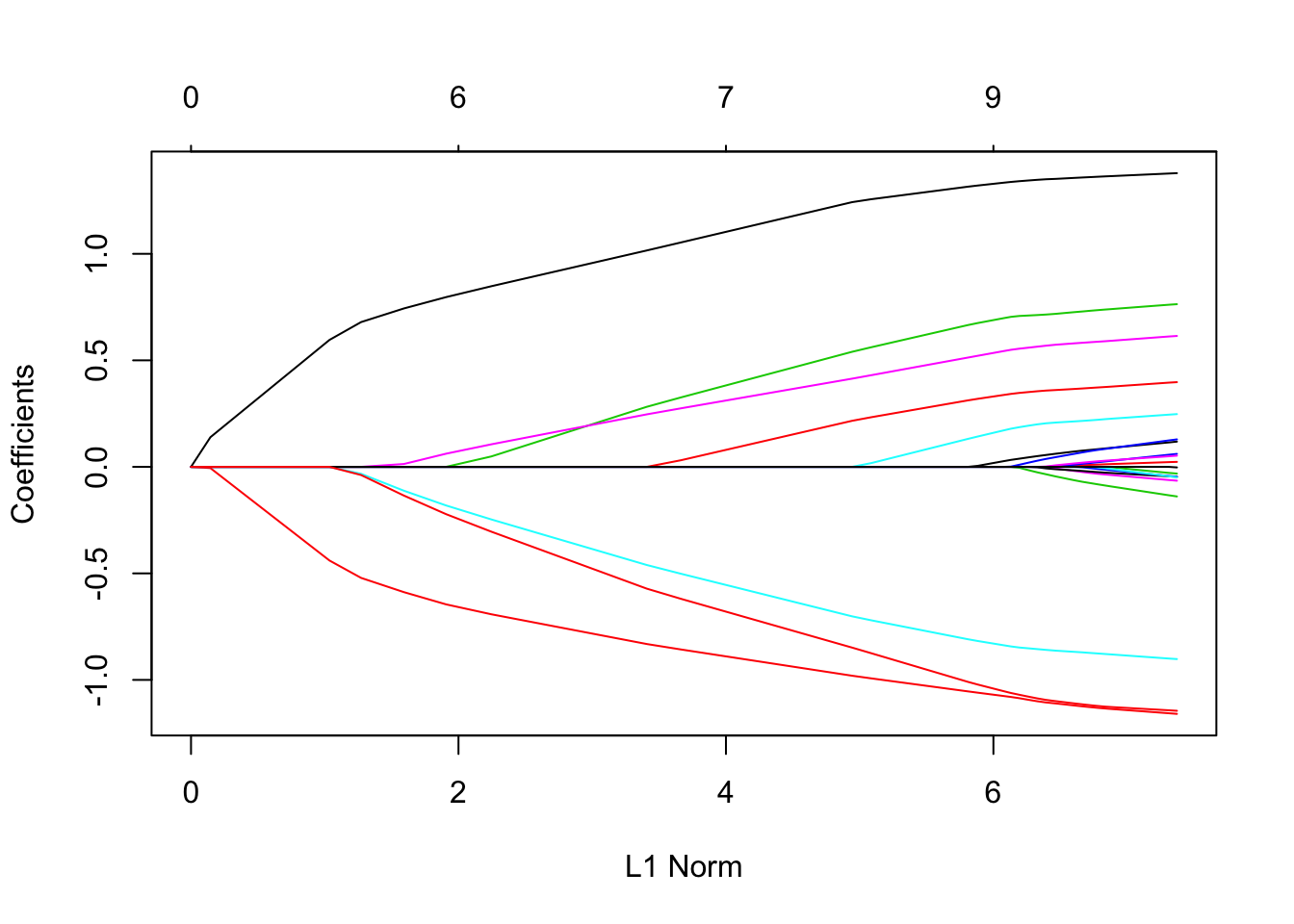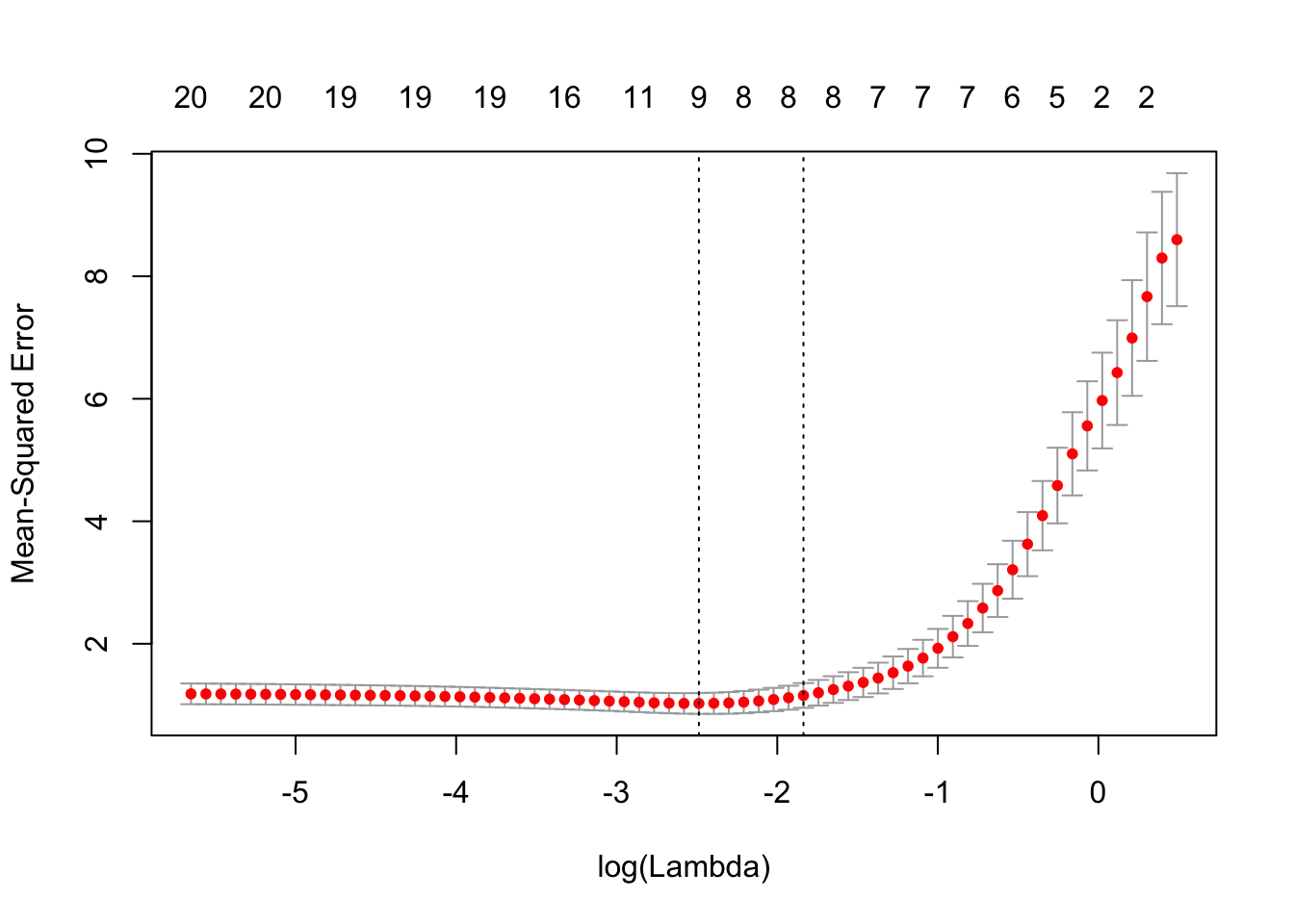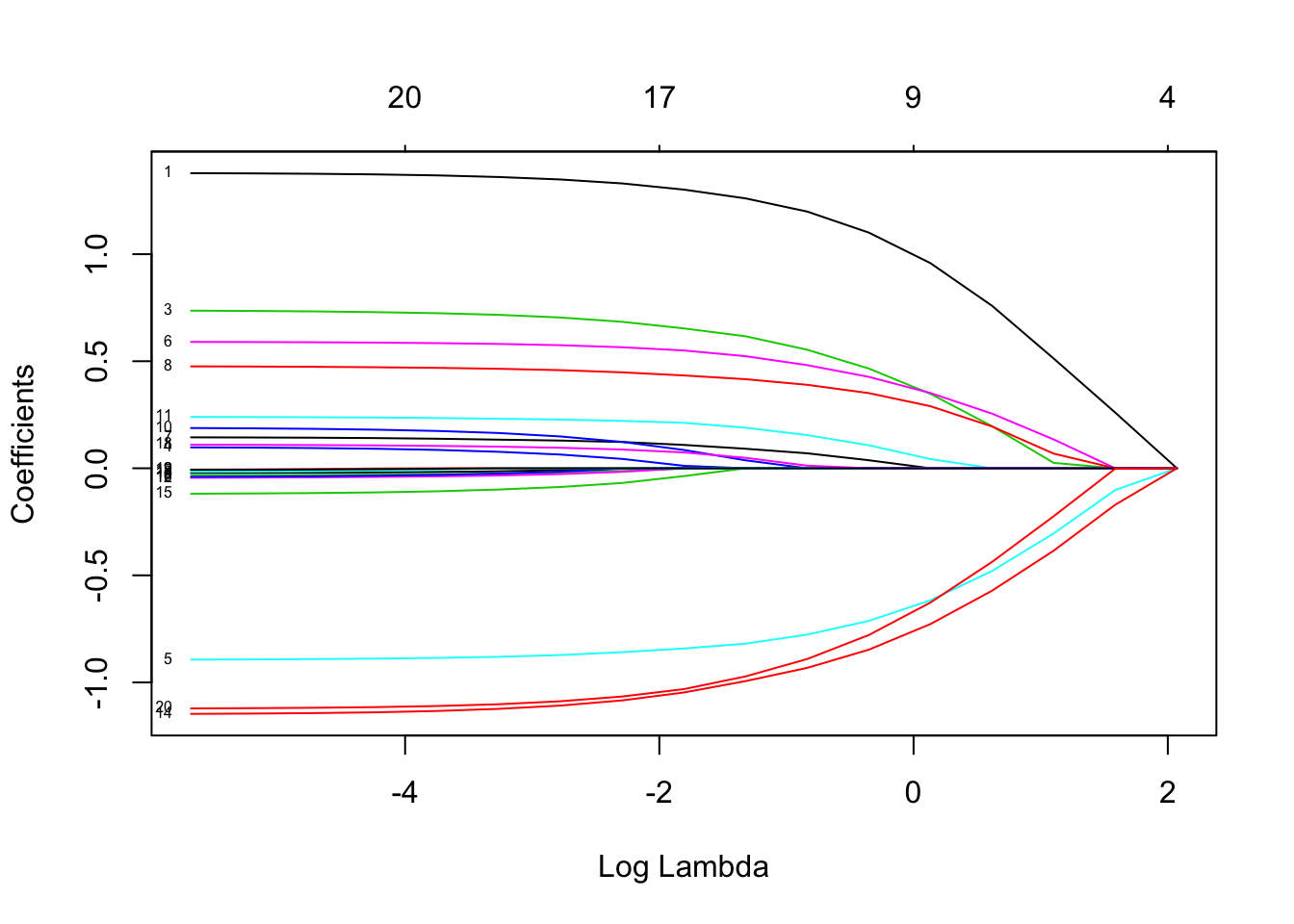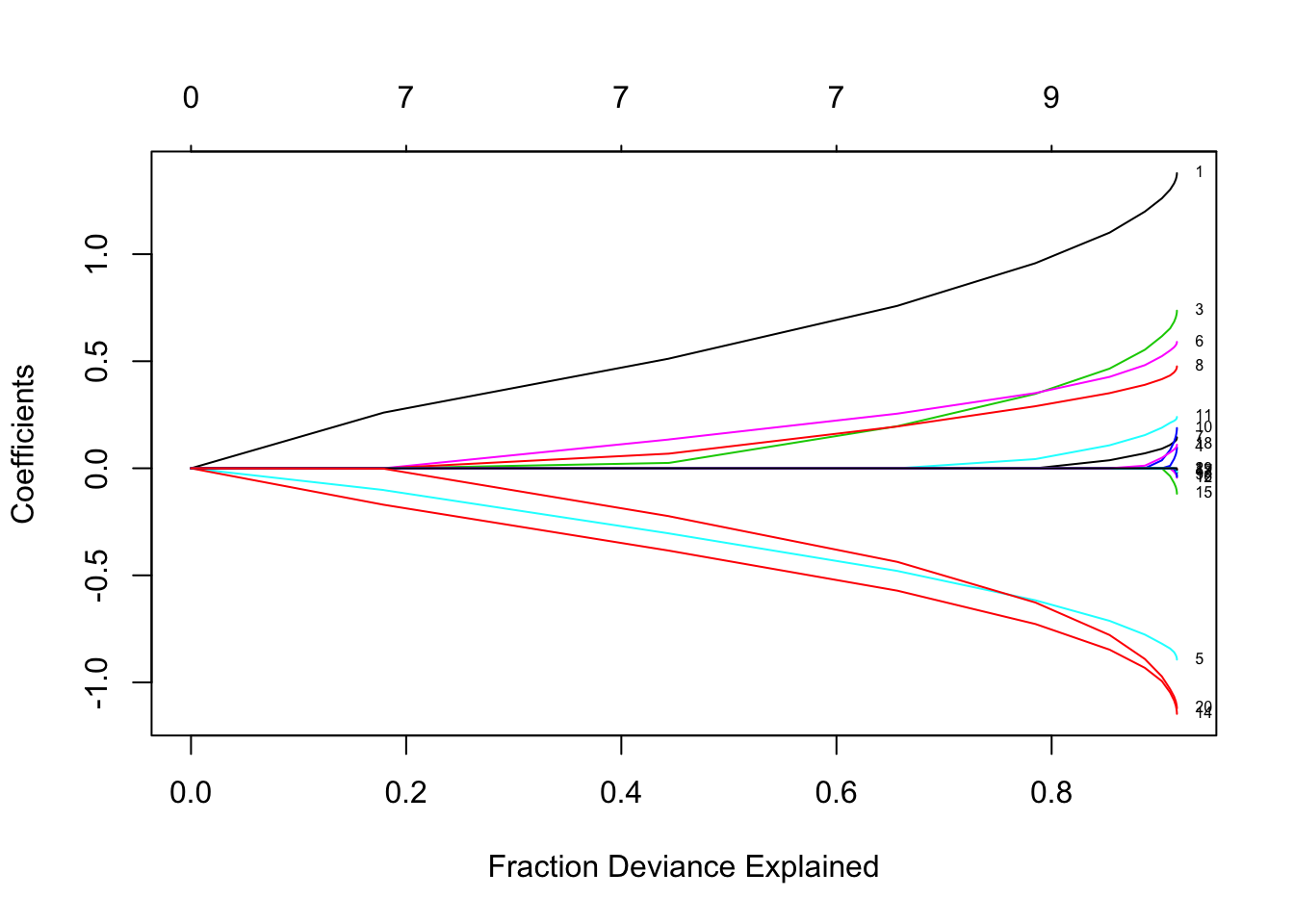# Glmnet Vignette

## Introduction

Glmnet is a package that fits a generalized linear model via penalized maximum likelihood. The regularization path is computed for the lasso or elasticnet penalty at a grid of values for the regularization parameter lambda. The algorithm is extremely fast, and can exploit sparsity in the input matrix x. It fits linear, logistic and multinomial, poisson, and Cox regression models. A variety of predictions can be made from the fitted models. It can also fit multi-response linear regression.

The authors of glmnet are Jerome Friedman, Trevor Hastie, Rob Tibshirani and Noah Simon, and the R package is maintained by Trevor Hastie. The matlab version of glmnet is maintained by Junyang Qian. This vignette describes the usage of glmnet in R.

glmnet solves the following problem $\min_{\beta_0,\beta} \frac{1}{N} \sum_{i=1}^{N} w_i l(y_i,\beta_0+\beta^T x_i) + \lambda\left[(1-\alpha)||\beta||_2^2/2 + \alpha ||\beta||_1\right],$ over a grid of values of $$\lambda$$ covering the entire range. Here $$l(y,\eta)$$ is the negative log-likelihood contribution for observation $$i$$; e.g. for the Gaussian case it is $$\frac{1}{2}(y-\eta)^2$$. The elastic-net penalty is controlled by $$\alpha$$, and bridges the gap between lasso ($$\alpha=1$$, the default) and ridge ($$\alpha=0$$). The tuning parameter $$\lambda$$ controls the overall strength of the penalty.

It is known that the ridge penalty shrinks the coefficients of correlated predictors towards each other while the lasso tends to pick one of them and discard the others. The elastic-net penalty mixes these two; if predictors are correlated in groups, an $$\alpha=0.5$$ tends to select the groups in or out together. This is a higher level parameter, and users might pick a value upfront, else experiment with a few different values. One use of $$\alpha$$ is for numerical stability; for example, the elastic net with $$\alpha = 1 - \epsilon$$ for some small $$\epsilon > 0$$ performs much like the lasso, but removes any degeneracies and wild behavior caused by extreme correlations.

The glmnet algorithms use cyclical coordinate descent, which successively optimizes the objective function over each parameter with others fixed, and cycles repeatedly until convergence. The package also makes use of the strong rules for efficient restriction of the active set. Due to highly efficient updates and techniques such as warm starts and active-set convergence, our algorithms can compute the solution path very fast.

The code can handle sparse input-matrix formats, as well as range constraints on coefficients. The core of glmnet is a set of fortran subroutines, which make for very fast execution.

The package also includes methods for prediction and plotting, and a function that performs K-fold cross-validation.

## Installation

Like many other R packages, the simplest way to obtain glmnet is to install it directly from CRAN. Type the following command in R console:

install.packages("glmnet", repos = "http://cran.us.r-project.org")

Users may change the repos options depending on their locations and preferences. Other options such as the directories where to install the packages can be altered in the command. For more details, see help(install.packages).

Here the R package has been downloaded and installed to the default directories.

Alternatively, users can download the package source at http://cran.r-project.org/web/packages/glmnet/index.html and type Unix commands to install it to the desired location.

## Quick Start

The purpose of this section is to give users a general sense of the package, including the components, what they do and some basic usage. We will briefly go over the main functions, see the basic operations and have a look at the outputs. Users may have a better idea after this section what functions are available, which one to choose, or at least where to seek help. More details are given in later sections.

First, we load the glmnet package:

library(glmnet)
## Loading required package: Matrix
## Loaded glmnet 1.9-9

The default model used in the package is the Guassian linear model or “least squares”, which we will demonstrate in this section. We load a set of data created beforehand for illustration. Users can either load their own data or use those saved in the workspace.

load("QuickStartExample.RData")

The command loads an input matrix x and a response vector y from this saved R data archive.

We fit the model using the most basic call to glmnet.

fit = glmnet(x, y)

“fit” is an object of class glmnet that contains all the relevant information of the fitted model for further use. We do not encourage users to extract the components directly. Instead, various methods are provided for the object such as plot, print, coef and predict that enable us to execute those tasks more elegantly.

We can visualize the coefficients by executing the plot function:

plot(fit)Each curve corresponds to a variable. It shows the path of its coefficient against the $$\ell_1$$-norm of the whole coefficient vector at as $$\lambda$$ varies. The axis above indicates the number of nonzero coefficients at the current $$\lambda$$, which is the effective degrees of freedom (df) for the lasso. Users may also wish to annotate the curves; this can be done by setting label = TRUE in the plot command.

A summary of the glmnet path at each step is displayed if we just enter the object name or use the print function:

print(fit)
##
## Call:  glmnet(x = x, y = y)
##
##       Df   %Dev  Lambda
##  [1,]  0 0.0000 1.63000
##  [2,]  2 0.0553 1.49000
##  [3,]  2 0.1460 1.35000
##  [4,]  2 0.2210 1.23000
##  [5,]  2 0.2840 1.12000
##  [6,]  2 0.3350 1.02000
##  [7,]  4 0.3900 0.93300
##  [8,]  5 0.4560 0.85000
##  [9,]  5 0.5150 0.77500
## [10,]  6 0.5740 0.70600
## [11,]  6 0.6260 0.64300
## [12,]  6 0.6690 0.58600
## [13,]  6 0.7050 0.53400
## [14,]  6 0.7340 0.48700
## [15,]  7 0.7620 0.44300
## [16,]  7 0.7860 0.40400
## [17,]  7 0.8050 0.36800
## [18,]  7 0.8220 0.33500
## [19,]  7 0.8350 0.30600
## [20,]  7 0.8460 0.27800
## [21,]  7 0.8560 0.25400
## [22,]  7 0.8630 0.23100
## [23,]  8 0.8710 0.21100
## [24,]  8 0.8770 0.19200
## [25,]  8 0.8820 0.17500
## [26,]  8 0.8860 0.15900
## [27,]  8 0.8900 0.14500
## [28,]  8 0.8930 0.13200
## [29,]  8 0.8960 0.12100
## [30,]  8 0.8980 0.11000
## [31,]  9 0.8990 0.10000
## [32,]  9 0.9010 0.09120
## [33,]  9 0.9020 0.08310
## [34,]  9 0.9030 0.07570
## [35,] 10 0.9040 0.06900
## [36,] 11 0.9050 0.06280
## [37,] 11 0.9060 0.05730
## [38,] 12 0.9070 0.05220
## [39,] 15 0.9080 0.04750
## [40,] 16 0.9090 0.04330
## [41,] 16 0.9090 0.03950
## [42,] 16 0.9100 0.03600
## [43,] 17 0.9100 0.03280
## [44,] 17 0.9110 0.02990
## [45,] 18 0.9110 0.02720
## [46,] 18 0.9110 0.02480
## [47,] 19 0.9120 0.02260
## [48,] 19 0.9120 0.02060
## [49,] 19 0.9120 0.01870
## [50,] 19 0.9120 0.01710
## [51,] 19 0.9130 0.01560
## [52,] 19 0.9130 0.01420
## [53,] 19 0.9130 0.01290
## [54,] 19 0.9130 0.01180
## [55,] 19 0.9130 0.01070
## [56,] 19 0.9130 0.00978
## [57,] 19 0.9130 0.00891
## [58,] 19 0.9130 0.00812
## [59,] 19 0.9130 0.00740
## [60,] 19 0.9130 0.00674
## [61,] 19 0.9130 0.00614
## [62,] 20 0.9130 0.00559
## [63,] 20 0.9130 0.00510
## [64,] 20 0.9130 0.00464
## [65,] 20 0.9130 0.00423
## [66,] 20 0.9130 0.00386
## [67,] 20 0.9130 0.00351

It shows from left to right the number of nonzero coefficients (Df), the percent (of null) deviance explained (%dev) and the value of $$\lambda$$ (Lambda). Although by default glmnet calls for 100 values of lambda the program stops early if %dev% does not change sufficently from one lambda to the next (typically near the end of the path.)

We can obtain the actual coefficients at one or more $$\lambda$$’s within the range of the sequence:

coef(fit,s=0.1)
## 21 x 1 sparse Matrix of class "dgCMatrix"
##                     1
## (Intercept)  0.150928
## V1           1.320597
## V2           .
## V3           0.675110
## V4           .
## V5          -0.817412
## V6           0.521437
## V7           0.004829
## V8           0.319416
## V9           .
## V10          .
## V11          0.142499
## V12          .
## V13          .
## V14         -1.059979
## V15          .
## V16          .
## V17          .
## V18          .
## V19          .
## V20         -1.021874

(why s and not lambda? In case later we want to allow one to specify the model size in other ways.) Users can also make predictions at specific $$\lambda$$’s with new input data:

nx = matrix(rnorm(10*20),10,20)
predict(fit,newx=nx,s=c(0.1,0.05))
##             1       2
##  [1,]  4.4641  4.7001
##  [2,]  1.7509  1.8513
##  [3,]  4.5207  4.6512
##  [4,] -0.6184 -0.6764
##  [5,]  1.7302  1.8451
##  [6,]  0.3565  0.3512
##  [7,]  0.2881  0.2662
##  [8,]  2.7776  2.8209
##  [9,] -3.7016 -3.7773
## [10,]  1.1546  1.1067

The function glmnet returns a sequence of models for the users to choose from. In many cases, users may prefer the software to select one of them. Cross-validation is perhaps the simplest and most widely used method for that task.

cv.glmnet is the main function to do cross-validation here, along with various supporting methods such as plotting and prediction. We still act on the sample data loaded before.

cvfit = cv.glmnet(x, y)

cv.glmnet returns a cv.glmnet object, which is “cvfit” here, a list with all the ingredients of the cross-validation fit. As for glmnet, we do not encourage users to extract the components directly except for viewing the selected values of $$\lambda$$. The package provides well-designed functions for potential tasks.

We can plot the object.

plot(cvfit)It includes the cross-validation curve (red dotted line), and upper and lower standard deviation curves along the $$\lambda$$ sequence (error bars). Two selected $$\lambda$$’s are indicated by the vertical dotted lines (see below).

We can view the selected $$\lambda$$’s and the corresponding coefficients. For example,

cvfit$lambda.min ##  0.08307 lambda.min is the value of $$\lambda$$ that gives minimum mean cross-validated error. The other $$\lambda$$ saved is lambda.1se, which gives the most regularized model such that error is within one standard error of the minimum. To use that, we only need to replace lambda.min with lambda.1se above. coef(cvfit, s = "lambda.min") ## 21 x 1 sparse Matrix of class "dgCMatrix" ## 1 ## (Intercept) 0.14936 ## V1 1.32975 ## V2 . ## V3 0.69096 ## V4 . ## V5 -0.83123 ## V6 0.53670 ## V7 0.02005 ## V8 0.33194 ## V9 . ## V10 . ## V11 0.16239 ## V12 . ## V13 . ## V14 -1.07081 ## V15 . ## V16 . ## V17 . ## V18 . ## V19 . ## V20 -1.04341 Note that the coefficients are represented in the sparse matrix format. The reason is that the solutions along the regularization path are often sparse, and hence it is more efficient in time and space to use a sparse format. If you prefer non-sparse format, pipe the output through as.matrix(). Predictions can be made based on the fitted cv.glmnet object. Let’s see a toy example. predict(cvfit, newx = x[1:5,], s = "lambda.min") ## 1 ## [1,] -1.3647 ## [2,] 2.5686 ## [3,] 0.5706 ## [4,] 1.9682 ## [5,] 1.4964 newx is for the new input matrix and s, as before, is the value(s) of $$\lambda$$ at which predictions are made. That is the end of glmnet 101. With the tools introduced so far, users are able to fit the entire elastic net family, including ridge regression, using squared-error loss. In the package, there are many more options that give users a great deal of flexibility. To learn more, move on to later sections. Back to Top ## Linear Regression Linear regression here refers to two families of models. One is gaussian, the Gaussian family, and the other is mgaussian, the multiresponse Gaussian family. We first discuss the ordinary Gaussian and the multiresponse one after that. ### Gaussian Family gaussian is the default family option in the function glmnet. Suppose we have observations $$x_i \in \mathbb{R}^p$$ and the responses $$y_i \in \mathbb{R}, i = 1, \ldots, N$$. The objective function for the Gaussian family is $\min_{(\beta_0, \beta) \in \mathbb{R}^{p+1}}\frac{1}{2N} \sum_{i=1}^N (y_i -\beta_0-x_i^T \beta)^2+\lambda \left[ (1-\alpha)||\beta||_2^2/2 + \alpha||\beta||_1\right],$ where $$\lambda \geq 0$$ is a complexity parameter and $$0 \leq \alpha \leq 1$$ is a compromise between ridge ($$\alpha = 0$$) and lasso ($$\alpha = 1$$). Coordinate descent is applied to solve the problem. Specifically, suppose we have current estimates $$\tilde{\beta_0}$$ and $$\tilde{\beta}_\ell$$ $$\forall j\in 1,]\ldots,p$$. By computing the gradient at $$\beta_j = \tilde{\beta}_j$$ and simple calculus, the update is $\tilde{\beta}_j \leftarrow \frac{S(\frac{1}{N}\sum_{i=1}^N x_{ij}(y_i-\tilde{y}_i^{(j)}),\lambda \alpha)}{1+\lambda(1-\alpha)},$ where $$\tilde{y}_i^{(j)} = \tilde{\beta}_0 + \sum_{\ell \neq j} x_{i\ell} \tilde{\beta}_\ell$$, and $$S(z, \gamma)$$ is the soft-thresholding operator with value $$\text{sign}(z)(|z|-\gamma)_+$$. This formula above applies when the x variables are standardized to have unit variance (the default); it is slightly more complicated when they are not. Note that for “family=gaussian”, glmnet standardizes $$y$$ to have unit variance before computing its lambda sequence (and then unstandardizes the resulting coefficients); if you wish to reproduce/compare results with other software, best to supply a standardized $$y$$ first (Using the “1/N” variance formula). glmnet provides various options for users to customize the fit. We introduce some commonly used options here and they can be specified in the glmnet function. • alpha is for the elastic-net mixing parameter $$\alpha$$, with range $$\alpha \in [0,1]$$. $$\alpha = 1$$ is the lasso (default) and $$\alpha = 0$$ is the ridge. • weights is for the observation weights. Default is 1 for each observation. (Note: glmnet rescales the weights to sum to N, the sample size.) • nlambda is the number of $$\lambda$$ values in the sequence. Default is 100. • lambda can be provided, but is typically not and the program constructs a sequence. When automatically generated, the $$\lambda$$ sequence is determined by lambda.max and lambda.min.ratio. The latter is the ratio of smallest value of the generated $$\lambda$$ sequence (say lambda.min) to lambda.max. The program then generated nlambda values linear on the log scale from lambda.max down to lambda.min. lambda.max is not given, but easily computed from the input $$x$$ and $$y$$; it is the smallest value for lambda such that all the coefficients are zero. For alpha=0 (ridge) lambda.max would be $$\infty$$; hence for this case we pick a value corresponding to a small value for alpha close to zero.) • standardize is a logical flag for x variable standardization, prior to fitting the model sequence. The coefficients are always returned on the original scale. Default is standardize=TRUE. For more information, type help(glmnet) or simply ?glmnet. As an example, we set $$\alpha = 0.2$$ (more like a ridge regression), and give double weights to the latter half of the observations. To avoid too long a display here, we set nlambda to 20. In practice, however, the number of values of $$\lambda$$ is recommended to be 100 (default) or more. In most cases, it does not come with extra cost because of the warm-starts used in the algorithm, and for nonlinear models leads to better convergence properties. fit = glmnet(x, y, alpha = 0.2, weights = c(rep(1,50),rep(2,50)), nlambda = 20) We can then print the glmnet object. print(fit) ## ## Call: glmnet(x = x, y = y, weights = c(rep(1, 50), rep(2, 50)), alpha = 0.2, nlambda = 20) ## ## Df %Dev Lambda ## [1,] 0 0.000 7.94000 ## [2,] 4 0.179 4.89000 ## [3,] 7 0.444 3.01000 ## [4,] 7 0.657 1.85000 ## [5,] 8 0.785 1.14000 ## [6,] 9 0.854 0.70300 ## [7,] 10 0.887 0.43300 ## [8,] 11 0.902 0.26700 ## [9,] 14 0.910 0.16400 ## [10,] 17 0.914 0.10100 ## [11,] 17 0.915 0.06230 ## [12,] 17 0.916 0.03840 ## [13,] 19 0.916 0.02360 ## [14,] 20 0.916 0.01460 ## [15,] 20 0.916 0.00896 ## [16,] 20 0.916 0.00552 ## [17,] 20 0.916 0.00340 This displays the call that produced the object fit and a three-column matrix with columns Df (the number of nonzero coefficients), %dev (the percent deviance explained) and Lambda (the corresponding value of $$\lambda$$). (Note that the digits option can used to specify significant digits in the printout.) Here the actual number of $$\lambda$$’s here is less than specified in the call. The reason lies in the stopping criteria of the algorithm. According to the default internal settings, the computations stop if either the fractional change in deviance down the path is less than $$10^{-5}$$ or the fraction of explained deviance reaches $$0.999$$. From the last few lines , we see the fraction of deviance does not change much and therefore the computation ends when meeting the stopping criteria. We can change such internal parameters. For details, see the Appendix section or type help(glmnet.control). We can plot the fitted object as in the previous section. There are more options in the plot function. Users can decide what is on the X-axis. xvar allows three measures: “norm” for the $$\ell_1$$-norm of the coefficients (default), “lambda” for the log-lambda value and “dev” for %deviance explained. Users can also label the curves with variable sequence numbers simply by setting label = TRUE. Let’s plot “fit” against the log-lambda value and with each curve labeled. plot(fit, xvar = "lambda", label = TRUE)Now when we plot against %deviance we get a very different picture. This is percent deviance explained on the training data. What we see here is that toward the end of the path this value are not changing much, but the coefficients are “blowing up” a bit. This lets us focus attention on the parts of the fit that matter. This will especially be true for other models, such as logistic regression. plot(fit, xvar = "dev", label = TRUE)We can extract the coefficients and make predictions at certain values of $$\lambda$$. Two commonly used options are: • s specifies the value(s) of $$\lambda$$ at which extraction is made. • exact indicates whether the exact values of coefficients are desired or not. That is, if exact = TRUE, and predictions are to be made at values of s not included in the original fit, these values of s are merged with object$lambda, and the model is refit before predictions are made. If exact=FALSE (default), then the predict function uses linear interpolation to make predictions for values of s that do not coincide with lambdas used in the fitting algorithm.

A simple example is:

any(fit$lambda == 0.5) ##  FALSE coef.exact = coef(fit, s = 0.5, exact = TRUE) coef.apprx = coef(fit, s = 0.5, exact = FALSE) cbind2(coef.exact, coef.apprx) ## 21 x 2 sparse Matrix of class "dgCMatrix" ## 1 1 ## (Intercept) 0.19657 0.199099 ## V1 1.17496 1.174650 ## V2 . . ## V3 0.52934 0.531935 ## V4 . . ## V5 -0.76126 -0.760959 ## V6 0.46627 0.468209 ## V7 0.06148 0.061927 ## V8 0.38049 0.380301 ## V9 . . ## V10 . . ## V11 0.14214 0.143261 ## V12 . . ## V13 . . ## V14 -0.91090 -0.911207 ## V15 . . ## V16 . . ## V17 . . ## V18 . 0.009197 ## V19 . . ## V20 -0.86099 -0.863117 The left column is for exact = TRUE and the right for FALSE. We see from the above that 0.01 is not in the sequence and that hence there are some difference, though not much. Linear interpolation is mostly enough if there are no special requirements. Users can make predictions from the fitted object. In addition to the options in coef, the primary argument is newx, a matrix of new values for x. The type option allows users to choose the type of prediction: * “link” gives the fitted values • “response” the sames as “link” for “gaussian” family. • “coefficients” computes the coefficients at values of s • “nonzero” retuns a list of the indices of the nonzero coefficients for each value of s. For example, predict(fit, newx = x[1:5,], type = "response", s = 0.05) ## 1 ## [1,] -0.9803 ## [2,] 2.2992 ## [3,] 0.6011 ## [4,] 2.3573 ## [5,] 1.7520 gives the fitted values for the first 5 observations at $$\lambda = 0.05$$. If multiple values of s are supplied, a matrix of predictions is produced. Users can customize K-fold cross-validation. In addition to all the glmnet parameters, cv.glmnet has its special parameters including nfolds (the number of folds), foldid (user-supplied folds), type.measure(the loss used for cross-validation): * “deviance” or “mse” uses squared loss • “mae” uses mean absolute error As an example, cvfit = cv.glmnet(x, y, type.measure = "mse", nfolds = 20) does 20-fold cross-validation, based on mean squared error criterion (default though). Parallel computing is also supported by cv.glmnet. To make it work, users must register parallel beforehand. We give a simple example of comparison here. require(doMC) ## Loading required package: doMC ## Loading required package: foreach ## Loading required package: iterators ## Loading required package: parallel registerDoMC(cores=2) X = matrix(rnorm(1e4 * 200), 1e4, 200) Y = rnorm(1e4) system.time(cv.glmnet(X, Y)) ## user system elapsed ## 3.591 0.103 3.724 system.time(cv.glmnet(X, Y, parallel = TRUE)) ## user system elapsed ## 4.318 0.391 2.700 As suggested from the above, parallel computing can significantly speed up the computation process especially for large-scale problems. Functions coef and predict on cv.glmnet object are similar to those for a glmnet object, except that two special strings are also supported by s (the values of $$\lambda$$ requested): * “lambda.1se”: the largest $$\lambda$$ at which the MSE is within one standard error of the minimal MSE. • “lambda.min”: the $$\lambda$$ at which the minimal MSE is achieved. cvfit$lambda.min
##  0.08307
coef(cvfit, s = "lambda.min")
## 21 x 1 sparse Matrix of class "dgCMatrix"
##                    1
## (Intercept)  0.14936
## V1           1.32975
## V2           .
## V3           0.69096
## V4           .
## V5          -0.83123
## V6           0.53670
## V7           0.02005
## V8           0.33194
## V9           .
## V10          .
## V11          0.16239
## V12          .
## V13          .
## V14         -1.07081
## V15          .
## V16          .
## V17          .
## V18          .
## V19          .
## V20         -1.04341
predict(cvfit, newx = x[1:5,], s = "lambda.min")
##            1
## [1,] -1.3647
## [2,]  2.5686
## [3,]  0.5706
## [4,]  1.9682
## [5,]  1.4964

Users can control the folds used. Here we use the same folds so we can also select a value for $$\alpha$$.

foldid=sample(1:10,size=length(y),replace=TRUE)
cv1=cv.glmnet(x,y,foldid=foldid,alpha=1)
cv.5=cv.glmnet(x,y,foldid=foldid,alpha=.5)
cv0=cv.glmnet(x,y,foldid=foldid,alpha=0)

There are no built-in plot functions to put them all on the same plot, so we are on our own here:

par(mfrow=c(2,2))
plot(cv1);plot(cv.5);plot(cv0)
plot(log(cv1$lambda),cv1$cvm,pch=19,col="red",xlab="log(Lambda)",ylab=cv1$name) points(log(cv.5$lambda),cv.5$cvm,pch=19,col="grey") points(log(cv0$lambda),cv0\$cvm,pch=19,col="blue")
legend("topleft",legend=c("alpha= 1","alpha= .5","alpha 0"),pch=19,col=c("red","grey","blue"))`